## Example 26.14 The Full Information Maximum Likelihood Method

This example shows how you can fully utilize all available information from the data when there is a high proportion of observations with random missing value. You use the full information maximum likelihood method for model estimation.

In Example 26.12, 32 students take six tests. These six tests are indicator measures of two ability factors: verbal and math. You conduct a confirmatory factor analysis in Example 26.12 based on a data set without any missing values. The path diagram for the confirmatory factor model is shown the following: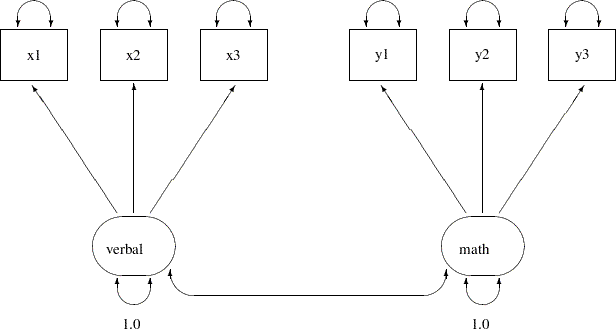Suppose now due to sickness or unexpected events, some students cannot take part in one of these tests. Now, the data test contains missing values at various locations, as indicated by the following DATA step:

```data missing;
input x1 x2 x3 y1 y2 y3;
datalines;
23   .  16  15  14  16
29  26  23  22  18  19
14  21   .  15  16  18
20  18  17  18  21  19
25  26  22   .  21  26
26  19  15  16  17  17
.  17  19   4   6   7
12  17  18  14  16   .
25  19  22  22  20  20
7  12  15  10  11   8
29  24   .  14  13  16
28  24  29  19  19  21
12   9  10  18  19   .
11   .  12  15  16  16
20  14  15  24  23  16
26  25   .  24  23  24
20  16  19  22  21  20
14   .  15  17  19  23
14  20  13  24   .   .
29  24  24  21  20  18
26   .  26  28  26  23
20  23  24  22  23  22
23  24  20  23  22  18
14   .  17   .  16  14
28  34  27  25  21  21
17  12  10  14  12  16
.   1  13  14  15  14
22  19  19  13  11  14
18  21   .  15  18  19
12  12  10  13  13  16
22  14  20  20  18  19
29  21  22  13  17   .
;
```

This data set is similar to the scores data set used in Example 26.12, except that some values are replaced at random with missing values. You can still fit the same confirmatory factor analysis model described in Example 26.12 to this data set by the default maximum likelihood (ML) method, as shown in the following statement:

```proc calis data=missing;
factor
verbal ---> x1-x3,
math   ---> y1-y3;
pvar
verbal = 1.,
math   = 1.;
run;
```

The data set, the number of observations, the model type, and analysis type are shown in the first table of Output 26.14.1. Although PROC CALIS reads all 32 records in the data set, only 16 of these records are used. The remaining 16 records contain at least one missing value in the tests. They are discarded from the analysis. Therefore, the maximum likelihood method only uses those 16 observations without missing values.

Output 26.14.1 Modeling Information of the CFA Model: Missing Data
 Confirmatory Factor Model With \Dataset{Missing} Data: ML FACTOR Model Specification

The CALIS Procedure
Covariance Structure Analysis: Model and Initial Values

Modeling Information
Data Set WORK.MISSING
N Records Used 16
N Obs 16
Model Type FACTOR
Analysis Covariances

Output 26.14.2 shows the parameter estimates.

Output 26.14.2 Parameter Estimates of the CFA Model: Missing Data
verbal math
x1
 5.1110 1.3110 3.8984 [_Parm1]
 0
x2
 5.6261 1.2561 4.4790 [_Parm2]
 0
x3
 4.8739 1.1410 4.2717 [_Parm3]
 0
y1
 0
 4.4529 0.8530 5.2205 [_Parm4]
y2
 0
 3.8562 0.8303 4.6444 [_Parm5]
y3
 0
 2.6338 0.7416 3.5513 [_Parm6]

Factor Covariance Matrix: Estimate/StdErr/t-value
verbal math
verbal
 1.0000
math
 1.0000

Error Variances
Variable Parameter Estimate Standard
Error
t Value

Most of the factor loading estimates shown in Output 26.14.2 are similar to those estimated from the data set without missing values, as shown in Output 26.12.4. The loading estimate of y3 on the math factor shows the largest discrepancy. With only half of the data used in the current estimation, this loading estimate is 2.6338 in the current analysis, while it is 3.7596 if no data were missing, as shown in Output 26.12.4. Another obvious difference between the two sets of results is that the standard error estimates for the loadings are consistently larger in the current analysis than in the analysis in Example 26.12 where there are no missing data. This is expected because you have only half of the data set available in the current analysis.

Similarly, the estimates for the factor covariance and error variances are mostly similar to those in the analysis with complete data, but the standard error estimates in the current analysis are consistently higher.

The maximum likelihood method, as implemented in PROC CALIS, deletes all observations with at least one missing value in the estimation. In a sense, the partially available information of these deleted observations is wasted. This greatly reduces the efficiency of the estimation, which results in higher standard error estimates.

To fully utilize all available information from the data set with the presence of missing values, you can use the full information maximum likelihood (FIML) method in PROC CALIS, as shown in the following statements:

```proc calis method=fiml data=missing;
factor
verbal ---> x1-x3,
math   ---> y1-y3;
pvar
verbal = 1.,
math   = 1.;
run;
```

In the PROC CALIS statement, you use METHOD=FIML to request the full information maximum likelihood method. Instead of deleting observations with missing values, the full information maximum likelihood method uses all available information in all observations. Output 26.14.3 shows some modeling information of the FIML estimation of the confirmatory factor model on the missing data.

Output 26.14.3 Modeling Information of the CFA Model with FIML: Missing Data
 Confirmatory Factor Model With Missing Data: FIML FACTOR Model Specification

The CALIS Procedure
Mean and Covariance Structures: Model and Initial Values

Modeling Information
Data Set WORK.MISSING
N Complete Records 16
N Incomplete Records 16
N Complete Obs 16
N Incomplete Obs 16
Model Type FACTOR
Analysis Means and Covariances

PROC CALIS shows you that the number of complete observations is 16 and the number of incomplete observations is 16 in the data set. All these observations are included in the estimation. The analysis type is 'Means and Covariances' because with full information maximum likelihood, the sample means have to be analyzed during the estimation.

For the full information maximum likelihood estimation, PROC CALIS outputs several tables to summarize the missing data patterns and statistics. Output 26.14.4 shows the proportions of data that are present for the variables, individually or jointly by pairs.

Output 26.14.4 Proportions of Data Present for the Variables: Missing Data
Proportions of Data Present for Means (Diagonal)
and Covariances (Off-Diagonal)
x1 x2 x3 y1 y2 y3
x1 0.9375
x2 0.7813 0.8438
x3 0.8125 0.7188 0.8750
y1 0.8750 0.8125 0.8125 0.9375
y2 0.9063 0.8125 0.8438 0.9063 0.9688
y3 0.8125 0.7188 0.7500 0.8125 0.8750 0.8750

 Average Proportion Coverage of Means 0.90625 0.816667

The diagonal elements of the table in Output 26.14.4 show the proportions of data coverage by each of the variables. The off-diagonal elements shows the proportions of joint data coverage by all possible pairs of variables. For example, the first diagonal element of the table shows that about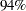of the observations have x1 values that are not missing. This percentage value is referred to as the proportion coverage for x1 or the proportion coverage for computing the means of x1. The off-diagonal element for x1 and x2 shows that about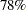of the observations have nonmissing values for both thier x1 and x2 values. This percentage value is referred to as the joint proportion coverage of x1 and x2 or the proportion coverage for computing the covariance between x1 and x2. The larger the coverage proportions this table shows, the more relative information the data contain for estimating the corresponding moments.

To summarize the proportion coverage, Output 26.14.4 shows that on average about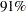of the data are nonmissing for computing the means, and about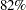of the data are nonmissing for computing the covariances.

Output 26.14.5 shows the lowest coverage proportions of the means and the covariances.

Output 26.14.5 Ranking the Lowest Coverage Proportions: Missing Data
Rank Order of the 3 Smallest Variable (Mean)
Coverages
Variable Coverage
x2 0.8438
x3 0.8750
y3 0.8750

Rank Order of the 7 Smallest Covariance Coverages
Var1 Var2 Coverage
x3 x2 0.7188
y3 x2 0.7188
y3 x3 0.7500
x2 x1 0.7813
x3 x1 0.8125
y1 x2 0.8125
y1 x3 0.8125

The first table of Output 26.14.5 shows that x2 has the lowest proportion coverage at about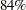, and x3 and y3 are the next at about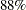. The second table of Output 26.14.5 shows that the joint proportion coverage by the x3-x2 pair and the y3-x2 pair are the lowest at about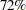, followed by the y3-x3 pair at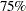. These two tables are useful to diagnose which variables most lack the information for estimation. For this data set, these tables show that estimation related to the moments of x2, x3, and y3 suffers the missing data problem the most. However, because the worst proportion coverage is still higher than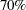, the missingness problem does not seem to be very serious based on percentage.

In Output 26.14.6, PROC CALIS outputs two tables that show an overall picture of the missing patterns in the data set.

Output 26.14.6 The Most Frequent Missing Patterns and Their Mean Profiles: Missing Data
Rank Order of the 5 Most Frequent Missing Patterns
Total Number of Distinct Patterns with Missing Values = 7
Pattern NVar
Miss
Freq Proportion Cumulative
1 x.xxxx 1 4 0.1250 0.1250
2 xx.xxx 1 4 0.1250 0.2500
3 xxxxx. 1 3 0.0938 0.3438
4 .xxxxx 1 2 0.0625 0.4063
5 xxxx.. 2 1 0.0313 0.4375

Means of the Nonmissing and the Most Frequent Missing Patterns
Variable Nonmissing
(N=16)
Missing Pattern
1
(N=4)
2
(N=4)
3
(N=3)
4
(N=2)
5
(N=1)
x1 21.75000 18.50000 21.75000 17.66667 . 14.00000
x2 19.37500 . 22.75000 15.66667 9.00000 20.00000
x3 19.31250 17.25000 . 16.66667 16.00000 13.00000
y1 19.00000 18.75000 17.00000 15.00000 9.00000 24.00000
y2 18.12500 18.75000 17.50000 17.33333 10.50000 .
y3 17.75000 19.50000 19.25000 . 10.50000 .

The first table of Output 26.14.6 shows that "x.xxxx" and "xx.xxx" are the two most frequent missing patterns in the data set. Each has a frequency of. An "x" in the missing pattern denotes a nonmissing value, while a "." denotes a missing value. Hence, the first pattern has all missing values for the second variable, and the second pattern has all missing values for the third variable. Each of these two missing patterns accounts for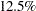of the total observations. Together, the five missing patterns shown in Output 26.14.6 account for about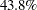of the total observations. The note after this table shows that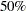of the total observations do not have any missing values.

To determine exactly which variables are missing in the missing patterns, it is useful to consult the second table in Output 26.14.6. In this table, the variable means of the most frequent missing patterns are shown, together with the variable means of the nonmissing pattern for comparisons. Missing means in this table show that the corresponding variables are not present in the missing patterns. For example, the column labeled "Nonmissing" is for the group of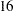observations that do not have any missing values. Each of the variable means is computed based onobservations. The next column labeled "1" is the first missing pattern that has four observations. The variable mean for x2 is missing for this missing pattern group, while each of the other variable means is computed based on four observations. Comparing these means with those in the nonmissing group, it shows that the means for x1, x3, and y1 in the first missing pattern are smaller than those in the nonmissing group, while the means for y2 and y3 are greater. This comparison does not seem to suggest any systematic bias in the means of the first missing pattern group.

However, the nonmissing means in the third missing pattern (the column labeled "3" do show a consistent downward bias, as compared with the means in the nonmissing group. This might mean that respondents with low scores in x1x3, y1, and y2 tend not to respond to y3 for some reason. Similarly, the fourth missing pattern shows a consistent downward bias in x2, x3, and y1y3. Whether these patterns suggest a systematic (or nonrandom) pattern of missingness must be judged in the substantive context. Nonetheless, the numerical results if Output 26.14.6 provide some insight on this matter.

The tables shown in Output 26.14.6 do not show all the missing patterns. In general, PROC CALIS shows only the most frequent or dominant missing patterns so that the output results are more focused. By default, if the total number of missing patterns in a data set is below six, then PROC CALIS shows all the missing patterns. If the total number of missing patterns is at least six, PROC CALIS shows up to 10 missing patterns provided that each of these missing patterns accounts for at least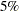of the total observations. The 10 missing patterns is the default maximum number of missing patterns to show, and theis the default proportion threshold for a missing pattern to display. You can override the default maximum number of missing patterns by the MAXMISSPAT= option and the proportion threshold by the TMISSPAT= option.

Output 26.14.7 shows the parameter estimates by the FIML estimation.

Output 26.14.7 Parameter Estimates of the CFA Model with FIML: Missing Data
verbal math
x1
 5.5003 1.0025 5.4867 [_Parm1]
 0
x2
 5.7134 0.9956 5.7385 [_Parm2]
 0
x3
 4.4417 0.7669 5.7918 [_Parm3]
 0
y1
 0
 4.9277 0.6798 7.2491 [_Parm4]
y2
 0
 4.1215 0.5716 7.2100 [_Parm5]
y3
 0
 3.3834 0.6145 5.5058 [_Parm6]

Factor Covariance Matrix: Estimate/StdErr/t-value
verbal math
verbal
 1.0000
math
 1.0000

Error Variances
Variable Parameter Estimate Standard
Error
t Value